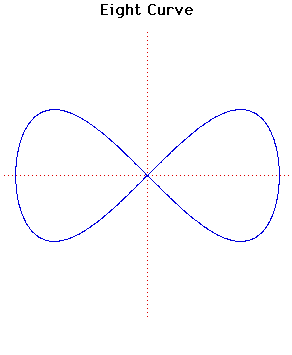# Curves

### Figure Eight CurveCartesian equation:
$x^{4} = a^{2}(x^{2}-y^{2})$
Polar equation:
$r^{2} = a^{2}\cos(2 \theta )\sec^{4}( \theta )$

### Description

This curve is also known as the Lemniscate of Gerono.

Other Web site:

Xah Lee

### Associated Curves

Definitions of the Associated curves